As described in my visibility post, my rules interpretation uses two kinds of visibility – visibility within a room, and line of sight.  Because of the anti door camping rule, line of sight is only used for corridors…  But even with this narrow scope, it’s still important!

Ray Casting in a Circle

My first attempt at line of sight was to implement ray casting in a circle.  I cast rays in degree increments (such as PI/16) from 0 to 2*PI.

For each ray, I step the line in increments such as dX = cos(theta) * scale, dY = sin(theta) * scale.  The scale factor can be adjusted.  Each time a step results in a new square space, I check if visibility is blocked.  For an orthogonal step, the check is simple.  For a diagonal step, I used the same implementation as described in my diagonal attacks post.

This first pass worked reasonable well.  However, I had some concerns…  The performance wasn’t really an issue (except as a theoretical exercise), but it’s worth mention that depending on your degree increment (such as PI/16), my algorithm was doing a lot of redundant checks.  The bigger concern I had was that the results didn’t exactly match the definition of line of sight that I wanted to use.

Here are some examples where I ended up having more squares be visible than I wanted.  The results are pretty good, but it doesn’t strictly match the desired line of sight definition.

Line of Sight Definition

The official game rules clearly define (albeit with the language “Rule of Thumb”) a definition of line of sight -  to see a square, you need to draw an unobstructed line from source square center to destination square center.

An astute observer will notice two strange things about the game’s one line of sight diagram.  It shows a two-wide horizontal corridor (or maybe the board is rotated 90 degrees?).  And the Elf’s right-side diagonal visible line crosses through the Wizard.  The rules probably mean it as an example of “touches a corner”.  The following similar example shows a line going through a goblin’s square.  The example is different than a 45 degree line that literally just “touches a corner” in the most minimal sense.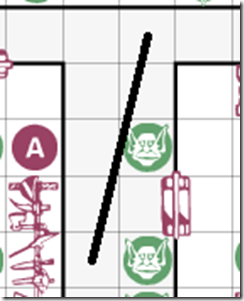Casting rays in a circle by a fixed angle of increments doesn’t do that.  The rays are cast in a direction based on an angle (by a constant angle increment about a circle).  This is different than casting a ray towards a destination square’s center.

Ray Casting to Target Square Centers

So instead of casting rays in angle increments, I moved on to an implementation that literally casts rays to target square centers.

The board only has 26*19 = 494 total squares, so simply casting rays to every single square on the board doesn’t seem that significant – because we only do it once after each move.

If we assume monsters don’t affect visibility, then it might make sense for each square to have a list of visible squares that is generated on map load.  Then we only need to update when something happens that affects visibility changes (eg a falling black trap is sprung).  If we include monsters, then we’d have to update relevant squares in our per-square visibility list whenever a monster moves (or dies).

Regardless of whether we maintain a per-square visibility list, we can reduce the number of target squares to check (for a given source square).  But one aspect we need to consider is how drastically the board can change.  Room placement can change due to expansion tiles.  But none of the official maps (or official tiles) change corridors in a way that’s relevant.

There aren’t any 3-wide corridors.  The longest 2-wide corridor is 7 squares long.  The 2-wide corridors are vertical.  So one idea, without special casing the logic based on the hero’s square, is to draw a rectangle around the hero that’s 7×13 (based on 3 dX, 6 dY) (that’s 91 squares).  Here’s an example: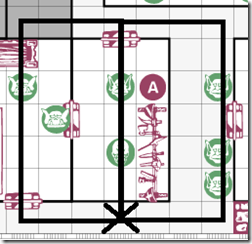Another idea is to take advantage of our particular rules interpretation.  For revealing a room, opening a door (or entering a room via “Pass Through Rock”) reveals the entire room.  Miniatures in the same room are always visible (for casting spells and ranged attacks).  With the anti door camping rule, we don’t allow seeing into other rooms for casting spells and ranged attacks.

So we can assume our line of sight algorithm only checks corridor squares.  That reduces the number of squares from 493 to 161 corridor squares (26+26+24+24+8+8+10+10+8+8+5+5-1).  We can also skip a corridor square unless it’s either (orthogonal) or (within 3 dX and 6 dY).  Here’s three scenarios where we need to check 3 dX or 6 dY: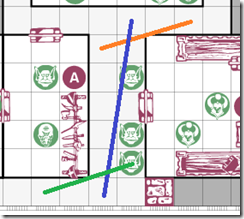Orthogonal squares can be handled by four simple ray casts, so let’s set these aside.  In the following examples, I counted how many non-orthogonal non-room squares are (within 3 dX and 6 dY).  The first has 11, the second has 14.

If we want to support custom maps with weird corridors, an easy way to extend this idea is just to include a wider bounding box than (3 dX, 6 dY).

One more easy trick – we can exclude squares marked as “dark”.

In summary…  Cast four orthogonal rays (East, North, West, South).  Cast rays to target square centers for squares that meet the following criteria – not part of a room, not a dark square, within 3 dX and 6 dY.

Center to Center means what it says

One case I had to debug was that with a Hero at (10, 18), I was seeing (12, 17) as visible.  I did expect to see (13, 17) as visible because a straight line goes to its center.  But a straight line from (10, 18) to (12, 17) is blocked by a wall.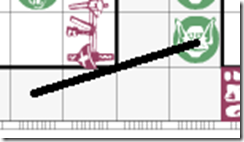The issue was that my algorithm was marking squares on the way to the target as visible.  In this example, when I cast a ray from (10, 18) to (13, 17), we travelled through (12, 17).  However, (12, 17) is not visible.  So I modified my code to only mark the target square as visible.

Implementation Corner Case

I ran into an interesting corner case with my implementation.  I got the following values.

xEnd, yEnd, iX, iY, fX, fY

HeroQuestLog: 0 17, 1 18 0.510000 17.509989 49
HeroQuestLog: 0 17, 1 17 0.500000 17.499989 50
HeroQuestLog: 0 17, 0 17 0.490000 17.489988 51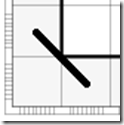In this example, we were checking for line of sight from (1, 18) to (0, 17).  Using the bit mask from my diagonal attacks post, this is a valid case (0110).  However, because my (dx dy) step is so small, we ended up visiting (1, 17).  Going from (1, 18) to (1, 17) is an orthogonal move though a wall – so it’s blocked.  This is the special case – "even if the line just touches a corner".

As a work-around, I simply skipped over these cases where the fractional part was 0.5.

Results – Reveal Corridors

I’ll close with a video showing line of sight used for reveal of corridors.  The video has white highlight squares that I used for debugging – I intentionally left these in the video to show what squares are being marked as visible.

The video shows some small quirks.  The orthogonal rays include room squares while the diagonal rays do not.  The double block only blocks one square instead of both squares.  I will do more tweaking, but overall it does the intended functionality – so I decided to post the video.  Fun stuff!

2016/07/16 Update: Touches a Corner, Manually Defined Lists

I was thinking about the game’s “touches a corner” example and how my square center to square center algorithm differs.  Here’s another idea on how to handle it.

There’s a small finite number of slopes in our taxicab geometry board with one-wide and two-wide corridors.  Because each quadrant (or octant) has symmetry, we can also divide this by four (or by eight).  1/2 can hold one list for (1 2) (-1 2) (1 –2) (-1 –2) (2 1) (-2 1) (2 –1) (-2 –1) because of the symmetry.  Also, (1 2) is the same as (2 4). because walking (2 4) is just walking (1 4) twice.

For each of these diagonal line slopes, we could simply hardcode which squares to check for obstruction.  With 3 dx 6 dy, the slopes in an octant are 1/2, 1/3, 1/4, 1/5, 1/6, 2/3, 2/5, 3/4, 3/5.  That’s only 9 total.

My current implementation of a ray cast takes tiny floating point steps and rounds to the nearest integer.  Instead of using floating point increments, we could walk a ray slope’s list of squares.

Consider the official example where the line touches the corner of the wizard’s square.  This example is 1/4 (dx = 4, dy = 1).  For 1/4, the list would be (x y) of (1 0) (3 1) (4 1).  Notice that we skip both (2 0) and (2 1).  This means if there is a wizard on both (2 0) and (2 1) then it would not obstruct.  But what if there is a block on both (2 0) and (2 1)?  Obviously a block on both (2 0) and (2 1) obstructs both reveal vision and spell vision.  So we’ll need some additional logic to differentiate between miniatures and blocks.

2016/07/16 Update: Use Area or Intersect Inscribed Circle

HeroQuest takes place on a 26×19 size board.  So even with custom maps that intentionally use wide corridors, or even an entire map that’s just a 26×19 corridor, there’s a limited number of slopes.  If we want to support a 26×19 corridor, we could just have more lists.  I’d have to check how many lists we’d need (and how tedious it would be to create those lists manually).

What if we want to support line of sight for a board bigger than 26×19 that’s made up entirely of corridors?  The real answer is we probably don’t, but for the sake of discussion…

Another idea is to determine whether a square is included in a ray cast based on the “area”.  When a ray is cast through a square, it cuts the square into two pieces.

Another idea is to inscribe a circle inside the square, and check whether the ray intersects that circle.  That will give slightly different results (than area) for very steep angles.

The results of each algorithm give slightly different results.  The results we prefer depends on our game design choices.  The algorithm I implemented for the above video includes any square that is touched by the ray – except corners touched at (0.5, 0.5) by 45 degree lines.  For now I’m sticking with that, but I may revise it later.